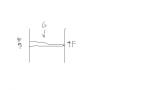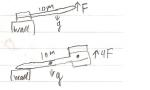# Centre of gravity and force

## Homework Statement

This is the case:

We gonna decide the centre of gravity to a flagstaff with length 10 m
We saved up the flagg staff in the thick end. We then need to use a vertical force F in the thin end to keep the flagstaff in horizontal balance. We then saves up the flagstaff in the thick end. Then we need to use a horizontal force on 4F in the thick end too keep the flagstaff in horizontal balance.
i) Count out where the centre of gravity to the flagstaff is.
ii) Count out the force F when the mass of the flagstaff is 250 kg.

Can anyone get me started ?

HallsofIvy
Homework Helper
I have no idea what this means:
We saved up the flagg staff in the thick end.

tiny-tim
Homework Helper
Hi Newton86!I'm confused.Can you give us the question in the original language … there'll probably be a lot of people on the forum who'll understand it!Hi Newton86!I'm confused.Can you give us the question in the original language … there'll probably be a lot of people on the forum who'll understand it!Ok:)

bildr.no/view/190755
Not sure i quite understand it myselftiny-tim
Homework Helper
ah … got it … !

We rest the thick end of the flagpole on something (a wall, maybe), and we weigh the other end, result F.

Then we rest the thin end of the flagpole on something, and we weigh the other end, result 4F.

Hint: take moments about the wall.one moore hint ? :P

tiny-tim
Homework Helper
ok … one more hint …

there are three forces acting on the flagpole …

draw a diagram …

draw a force N where it rests on the wall, its weight W vertically through the centre of gravity, and the force F (or 4F) vertically at the other end.

You don't know what N is, and you don't want to know, so you eliminate N by taking moments about the wall.

Have a go … !Like this ? Is there moore forces ?

tiny-tim
Homework Helper
(btw, it's "more", not "moore")

Hi Newton86!Nooo …

That's a really bad diagram … what are those two vertical lines on either side? … and you should have the arrows starting (or ending) at the point of application of the force … and you haven't marked the length of the flagpole or the postion of the centre of gravity.

And you need two diagrams … one with the wall at the thick end and F at the thin end, the other with the wall at the thin end and 4F at the thick end.

It is very very important that you get used to making clear accurate diagrams. If you don't, you may make mistakes, or even not be able to answer the question at all.ok … draw one of the diagrams, and then take moments about the wall.hmm Not sure i understand it quite but I tryed again.Do I know where the center of the gravity is ?

I dont get this Iv searched for other examples too but no one uses forces =|

tiny-tim
Homework Helper
Hi Newton86!Now that's a much better diagram!Do I know where the center of the gravity is ?

No … but that's what the question asks you to find … so the standard trick is to give it a name, like x, and put x in the diagram.

ok … now what, in terms of W and x, is the moment of the weight about the wall?

and what is the moment of the F force about the wall?Hi Newton86!Now that's a much better diagram!No … but that's what the question asks you to find … so the standard trick is to give it a name, like x, and put x in the diagram.

ok … now what, in terms of W and x, is the moment of the weight about the wall?

and what is the moment of the F force about the wall?I would like to know that. I dont find any formulas ( used all day )How can a noob get such a tasktiny-tim
Homework Helper
cheer up!Moment about a point = force times distance (that's the distance from the point to the line of the force).

In this case, the distances of the three forces from the wall are 0, x, and 10 in the first diagram, and … ? in the second diagram.

So the total moment about the top of the wall is 0 + x.W - 10.F in the first diagram, and … ? in the second diagram.

When there is no turning, the total moment about any point must be 0.

(is any of this familiar?)

So … ?Ah This looks familiar too vectors.In this case, the distances of the three forces from the wall are 0, x, and 10 in the first diagram, and the same distance in 0, x, 10 ? in the second diagram.

So the total moment about the top of the wall is 0 + x.W - 10.F in the first diagram, and 0 + x.W - 10.4F ( -40F? )? in the second diagram.

( 0, x, -10 )( 0,x,-40) ?

wild guess is the the center of gravity is 7,07m from the thin end

tiny-tim
Homework Helper
Hi Newton86!In this case, the distances of the three forces from the wall are 0, x, and 10 in the first diagram, and the same distance in 0, x, 10 ? in the second diagram.

oooh… you didn't put x into your diagrams, did you?

If you had, you'd have seen that it's 10 - x in the second diagram … right?

That's why good diagrams are so important!

So the answer is … ?ye I was unsure about that. But I dont see how I can use this too find the center of gravity
and What about the other diagram was it wrong too?

Last edited:
tiny-tim
Homework Helper
Hi Newton86!Your second formula would have been right, except that it shouldn't have had x.

Get it right, put the two formulas equal to zero (you do understand why, don't you?), and then solve the two equations!Could you set them up correctly ?maybe I will understand a bit moore caus im a bit confused on thistiny-tim
Homework Helper
No!

Show us what you can do … then we can see where the problem is!Ok Im thinking (0,x,10)(10-x,0) =0

tiny-tim
Homework Helper
Ok Im thinking (0,x,10)(10-x,0) =0

But that doesn't even mean anything!(they're not vectors … and even if they were, how would you combine a 3-term vector with a 3-term one?)

Go back to:
So the total moment about the top of the wall is 0 + x.W - 10.F in the first diagram …

Now, (as I told you) that was right!.

So the total moment about the wall (for the first diagram) is x.W - 10.F.

And there is no turning, so this has to be zero (do you understand why that is … you haven't said … ?), and so:

x.W = 10.F.​

Now do the same thing for the second diagram … you should get a similar equation … what is it … ?no I cant say I know why it has to be equal to zero.:shy:
But momentum was force times length from the point to the force so I think the second diagram then must be:
x.W = 10*4 = 40.F ?

tiny-tim
Then tell me if you understand why the moments have to add to zero.